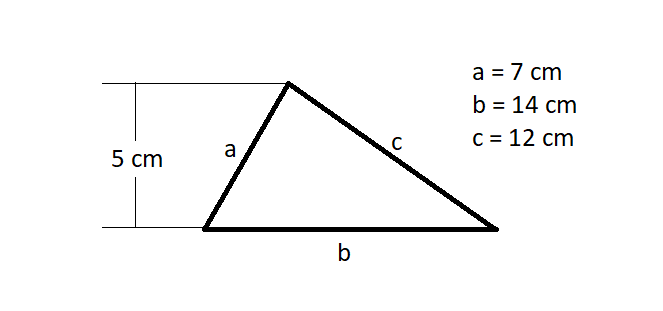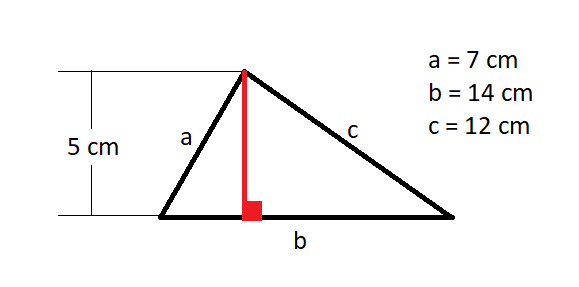# Help Zone

### Student Question

How to calculate the area and the perimeter of this triangle:Thank you!

Mathematics{t c="richEditor.description.title"} {t c="richEditor.description.paragraphMenu"} {t c="richEditor.description.inlineMenu"} {t c="richEditor.description.embed"}

## Explanations (1)

•Explanation from AlloprofExplanation from Alloprof

This Explanation was submitted by a member of the Alloprof team.

Hello again!

The perimeter is the measure of the outline of a figure. By adding all the sides, we can find it.

Area is a measure of the area occupied by an object in 2 dimensions.

So the perimeter of this triangle is just side a + side b + side c.

Regarding the area of ​​a triangle, it is its base multiplied by its height ÷ by 2. You should know that the base of a triangle is always perpendicular to its height. So in your picture, the height corresponds to this (in red):So the height of this triangle is 5 cm.

Now you are able to calculate your perimeter and area.

Have a nice day.

KH